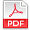## Scientific Review

Online ISSN: 2412-2599
Print ISSN: 2413-8835

Frequency: Monthly

# Archives

### Volume 4 Number 4 April 2018

#### Anekwe's Corrections on the Negative Binomial ExpansionPDF

Authors: Uchenna Okwudili Anekwe
Pages: 26-33
Abstract
For so many years now a lot of scientist have used the series of positive binomial expansion to solve that of Negative binomial expansion, positive fractional binomial expansion and Negative fractional binomial expansion which was generated/derived using Maclaurin series to derive the series of Negative binomial expansion, positive fractional binomial expansion and Negative fractional binomial expansion just as it was used to provide answers to positive binomial expansion but fails for All the other expansion due to a deviation made. This Manuscript contains the correct solution/answers to Negative binomial expansions with proofs through worked examples, with other forms of solving Negative binomial expansion just as in the case of Pascal’s triangle in positive binomial expansion, in Negative binomial expansion it is called Anekwe’s triangle and other methods like the combination method of solving Negative binomial expansion.